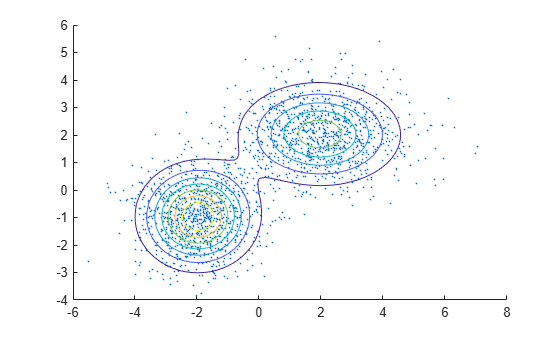# cluster

Construct clusters from Gaussian mixture distribution

## Syntax

``idx = cluster(gm,X)``
``[idx,nlogL] = cluster(gm,X)``
``[idx,nlogL,P] = cluster(gm,X)``
``[idx,nlogL,P,logpdf] = cluster(gm,X)``
``[idx,nlogL,P,logpdf,d2] = cluster(gm,X)``

## Description

example

````idx = cluster(gm,X)` partitions the data in `X` into k clusters determined by the k Gaussian mixture components in `gm`. The value in `idx(i)` is the cluster index of observation `i` and indicates the component with the largest posterior probability given the observation `i`.```
````[idx,nlogL] = cluster(gm,X)` also returns the negative loglikelihood of the Gaussian mixture model `gm` given the data `X`.```
````[idx,nlogL,P] = cluster(gm,X)` also returns the posterior probabilities of each Gaussian mixture component in `gm` given each observation in `X`.```
````[idx,nlogL,P,logpdf] = cluster(gm,X)` also returns a logarithm of the estimated probability density function (pdf) evaluated at each observation in `X`.```
````[idx,nlogL,P,logpdf,d2] = cluster(gm,X)` also returns the squared Mahalanobis distance of each observation in `X` to each Gaussian mixture component in `gm`.```

## Examples

collapse all

Generate random variates that follow a mixture of two bivariate Gaussian distributions by using the `mvnrnd` function. Fit a Gaussian mixture model (GMM) to the generated data by using the `fitgmdist` function. Then, use the `cluster` function to partition the data into two clusters determined by the fitted GMM components.

Define the distribution parameters (means and covariances) of two bivariate Gaussian mixture components.

```mu1 = [2 2]; % Mean of the 1st component sigma1 = [2 0; 0 1]; % Covariance of the 1st component mu2 = [-2 -1]; % Mean of the 2nd component sigma2 = [1 0; 0 1]; % Covariance of the 2nd component```

Generate an equal number of random variates from each component, and combine the two sets of random variates.

```rng('default') % For reproducibility r1 = mvnrnd(mu1,sigma1,1000); r2 = mvnrnd(mu2,sigma2,1000); X = [r1; r2];```

The combined data set `X` contains random variates following a mixture of two bivariate Gaussian distribution.

Fit a two-component GMM to `X`.

`gm = fitgmdist(X,2);`

Plot `X` by using `scatter`. Visualize the fitted model `gm` by using `pdf` and `fcontour`.

```figure scatter(X(:,1),X(:,2),10,'.') % Scatter plot with points of size 10 hold on gmPDF = @(x,y) arrayfun(@(x0,y0) pdf(gm,[x0 y0]),x,y); fcontour(gmPDF,[-6 8 -4 6])```Partition the data into clusters by passing the fitted GMM and the data to `cluster`.

`idx = cluster(gm,X);`

Use `gscatter` to create a scatter plot grouped by `idx`.

```figure; gscatter(X(:,1),X(:,2),idx); legend('Cluster 1','Cluster 2','Location','best');```## Input Arguments

collapse all

Gaussian mixture distribution, also called Gaussian mixture model (GMM), specified as a `gmdistribution` object.

You can create a `gmdistribution` object using `gmdistribution` or `fitgmdist`. Use the `gmdistribution` function to create a `gmdistribution` object by specifying the distribution parameters. Use the `fitgmdist` function to fit a `gmdistribution` model to data given a fixed number of components.

Data, specified as an n-by-m numeric matrix, where n is the number of observations and m is the number of variables in each observation.

To provide meaningful clustering results, `X` must come from the same population as the data used to create `gm`.

If a row of `X` contains `NaNs`, then `cluster` excludes the row from the computation. The corresponding value in `idx`, `P`, `logpdf`, and `d2` is `NaN`.

Data Types: `single` | `double`

## Output Arguments

collapse all

Cluster index, returned as an n-by-1 positive integer vector, where n is the number of observations in `X`.

`idx(i)` is the cluster index of observation `i` and indicates the Gaussian mixture component with the largest posterior probability given the observation `i`.

Negative loglikelihood value of the Gaussian mixture model `gm` given the data `X`, returned as a numeric value.

Posterior probability of each Gaussian mixture component in `gm` given each observation in `X`, returned as an n-by-k numeric vector, where n is the number of observations in `X` and k is the number of mixture components in `gm`.

`P(i,j)` is the posterior probability of the `j`th Gaussian mixture component given observation `i`, Probability(component `j` | observation `i`).

Logarithm of the estimated pdf, evaluated at each observation in `X`, returned as an n-by-1 numeric vector, where n is the number of observations in `X`.

`logpdf(i)` is the logarithm of the estimated pdf at observation `i`. The `cluster` function computes the estimated pdf by using the likelihood of each component given each observation and the component probabilities.

`$\text{logpdf}\left(i\right)=\mathrm{log}\sum _{j=1}^{k}L\left({C}_{j}|{O}_{i}\right)\text{P}\left({\text{C}}_{j}\right),$`

where L(Cj|Oj) is the likelihood of component `j` given observation `i`, and P(Cj) is the probability of component `j`. The `cluster` function computes the likelihood term by using the multivariate normal pdf of the `j`th Gaussian mixture component evaluated at observation `i`. The component probabilities are the mixing proportions of mixture components, the `ComponentProportion` property of `gm`.

Squared Mahalanobis distance of each observation in `X` to each Gaussian mixture component in `gm`, returned as an n-by-k numeric matrix, where n is the number of observations in `X` and k is the number of mixture components in `gm`.

`d2(i,j)` is the squared distance of observation `i` to the `j`th Gaussian mixture component.